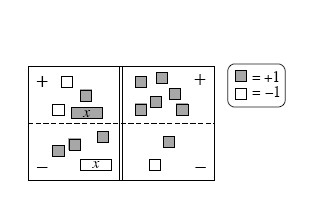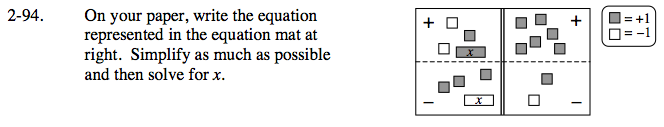### Home > CAAC > Chapter 2 > Lesson 2.2.1 > Problem2-94

2-94.

On your paper, write the equation represented in the equation mat below. Simplify as much as possible and then solve for x. 2-94 HW eTool (CPM). Homework Help ✎Move all tiles in the subtraction region to the addition region by flipping the tiles from positive to negative, and remove any zero pairs. Write the equation represented.

2x − 4 = 6 Solve for x.

Use the eTool below to help solve the problem.
Click the link at right for the full version of the eTool: AC 2-94 HW eTool